Ex﻿

# 2. Force & Laws Of Motion

#### Density

Density Formula →SI unit of density is kg/m3 and CGS unit of density is g /cm3
1 gm/cm3= 1000 kg/m3
Different substances have different densities which gets affected by temperature.
Respective density of a substance decreases on heating due to the expansion of the substance.
Water has anomalous expansion. When water is cooled at 4ºC, its volume decreases but on further cooling its volume starts increasing.
→ the density of water is maximum at 4ºC.

Relative density
Relative density of a substance
=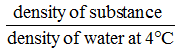==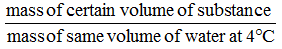=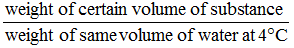Unit of Relative Density
It has no units.
Note : Density of water in CGS system is 1 gm/cm3.
Relative Density for solids
i.e.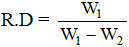Where W1 = weight of solid body in air
W2 = weight of solid body in water
Relative Density for liquids
i.e R.D. =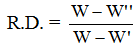Where.
W' = weight of the body fully immersed in water
W" = weight of the body fully immersed in liquid.
W = weight of the body in air

If you want to give information about online courses to other students, then share it with more and more on Facebook, Twitter, Google Plus. The more the shares will be, the more students will benefit. The share buttons are given below for your convenience.
×

#### NTSE Physics (Class X)

• Electricity
• Magnetic Effect of Curent
• Light Reflection
• Light Refraction
• Human Eye & Colourful World
• Source of Energy
• Motion

#### NTSE Physics (Class IX)

• Force & laws of motion
• Work and Energy
• Gravitation
• Sound

SHOW CHAPTERS